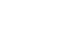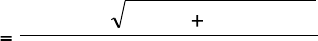Study Guides (390,000)
US (220,000)
KSU (400)
BUS (10)
Final

# BUS 10123 Chapter Notes - Chapter 6: Autoregressive Model, Partial Autocorrelation Function, AutocorrelationExam

Department
Course Code
BUS 10123
Professor
Eric Von Hendrix
Study Guide
Final

This preview shows pages 1-3. to view the full 11 pages of the document.1
1. Autoregressive models specify the current value of a series yt as a function of its
previous p values and the current value an error term, ut, while moving average
models specify the current value of a series yt as a function of the current and
previous q values of an error term, ut. AR and MA models have different
characteristics in terms of the length of their “memories”, which has implications for
the time it takes shocks to yt to die away, and for the shapes of their autocorrelation
and partial autocorrelation functions.
2. ARMA models are of particular use for financial series due to their flexibility. They
are fairly simple to estimate, can often produce reasonable forecasts, and most
importantly, they require no knowledge of any structural variables that might be
required for more “traditional” econometric analysis. When the data are available at
high frequencies, we can still use ARMA models while exogenous “explanatory”
variables (e.g. macroeconomic variables, accounting ratios) may be unobservable at
any more than monthly intervals at best.
3. yt = yt-1 + ut (1)
yt = 0.5 yt-1 + ut (2)
yt = 0.8 ut-1 + ut (3)
(a) The first two models are roughly speaking AR(1) models, while the last is an
MA(1). Strictly, since the first model is a random walk, it should be called an
ARIMA(0,1,0) model, but it could still be viewed as a special case of an
autoregressive model.
(b) We know that the theoretical acf of an MA(q) process will be zero after q lags, so
the acf of the MA(1) will be zero at all lags after one. For an autoregressive process,
the acf dies away gradually. It will die away fairly quickly for case (2), with each
successive autocorrelation coefficient taking on a value equal to half that of the
previous lag. For the first case, however, the acf will never die away, and in theory
will always take on a value of one, whatever the lag.
Turning now to the pacf, the pacf for the first two models would have a large
positive spike at lag 1, and no statistically significant pacf’s at other lags. Again, the
unit root process of (1) would have a pacf the same as that of a stationary AR
process. The pacf for (3), the MA(1), will decline geometrically.
(c) Clearly the first equation (the random walk) is more likely to represent stock
prices in practice. The discounted dividend model of share prices states that the
current value of a share will be simply the discounted sum of all expected future
dividends. If we assume that investors form their expectations about dividend
payments rationally, then the current share price should embody all information that
is known about the future of dividend payments, and hence today’s price should
only differ from yesterday’s by the amount of unexpected news which influences
dividend payments.

Only pages 1-3 are available for preview. Some parts have been intentionally blurred.

Thus stock prices should follow a random walk. Note that we could apply a similar
rational expectations and random walk model to many other kinds of financial series.
If the stock market really followed the process described by equations (2) or (3), then
we could potentially make useful forecasts of the series using our model. In the
latter case of the MA(1), we could only make one-step ahead forecasts since the
“memory” of the model is only that length. In the case of equation (2), we could
potentially make a lot of money by forming multiple step ahead forecasts and
trading on the basis of these.
Hence after a period, it is likely that other investors would spot this potential
opportunity and hence the model would no longer be a useful description of the
data.
(d) See the book for the algebra. This part of the question is really an extension of
the others. Analysing the simplest case first, the MA(1), the “memory” of the
process will only be one period, and therefore a given shock or “innovation”, ut, will
only persist in the series (i.e. be reflected in yt) for one period. After that, the effect
of a given shock would have completely worked through.
For the case of the AR(1) given in equation (2), a given shock, ut, will persist
indefinitely and will therefore influence the properties of yt for ever, but its effect
upon yt will diminish exponentially as time goes on.
In the first case, the series yt could be written as an infinite sum of past shocks, and
therefore the effect of a given shock will persist indefinitely, and its effect will not
diminish over time.
4. (a) Box and Jenkins were the first to consider ARMA modelling in this logical
and coherent fashion. Their methodology consists of 3 steps:
Identification - determining the appropriate order of the model using
graphical procedures (e.g. plots of autocorrelation functions).
Estimation - of the parameters of the model of size given in the first stage.
This can be done using least squares or maximum likelihood, depending on
the model.
Diagnostic checking - this step is to ensure that the model actually estimated
is “adequate”. B & J suggest two methods for achieving this:
- Overfitting, which involves deliberately fitting a model larger than that
suggested in step 1 and testing the hypothesis that all the additional
coefficients can jointly be set to zero.
- Residual diagnostics. If the model estimated is a good description of the
data, there should be no further linear dependence in the residuals of the
estimated model. Therefore, we could calculate the residuals from the
estimated model, and use the Ljung-Box test on them, or calculate their acf. If
either of these reveal evidence of additional structure, then we assume that
the estimated model is not an adequate description of the data.

Only pages 1-3 are available for preview. Some parts have been intentionally blurred.If the model appears to be adequate, then it can be used for policy analysis
and for constructing forecasts. If it is not adequate, then we must go back to
stage 1 and start again!
(b) The main problem with the B & J methodology is the inexactness of the
identification stage. Autocorrelation functions and partial autocorrelations
for actual data are very difficult to interpret accurately, rendering the whole
procedure often little more than educated guesswork. A further problem
concerns the diagnostic checking stage, which will only indicate when the
proposed model is “too small” and would not inform on when the model
proposed is “too large”.
(c) We could use Akaike’s or Schwarz’s Bayesian information criteria. Our
objective would then be to fit the model order that minimises these.
We can calculate the value of Akaike’s (AIC) and Schwarz’s (SBIC) Bayesian
information criteria using the following respective formulae
AIC = ln ( ) + 2k/T
SBIC = ln ( ) + k ln(T)/T
The information criteria trade off an increase in the number of parameters
and therefore an increase in the penalty term against a fall in the RSS,
implying a closer fit of the model to the data.
5. The best way to check for stationarity is to express the model as a lag polynomial
in yt.
y y y u
t t t t
= + +
฀ ฀
0 803 0 682
1 2
. .
Rewrite this as
y L L u
t t
( . . )1 0 803 0 682 2
฀ ฀ =
We want to find the roots of the lag polynomial
( . . )1 0 803 0 682 0
2
฀ ฀ =L L
and
determine whether they are greater than one in absolute value. It is easier (in my
opinion) to rewrite this formula (by multiplying through by -1/0.682, using z for the
characteristic equation and rearranging) as
z2 + 1.177 z - 1.466 = 0
Using the standard formula for obtaining the roots of a quadratic equation,
= 0.758 or 1.934
Since ALL the roots must be greater than one for the model to be stationary, we
conclude that the estimated model is not stationary in this case.
6. Using the formulae above, we end up with the following values for each criterion
and for each model order (with an asterisk denoting the smallest value of the
information criterion in each case).